Numerical Simulation of the Behavior for a Vortex Ring Convecting along the Axis of a Rotating Field | OMICS International
Fluid Mechanics: Open Access

# Numerical Simulation of the Behavior for a Vortex Ring Convecting along the Axis of a Rotating Field

Tomomi Uchiyama1*, Masato Nakano2 and Tomohiro Degawa3

1EcoTopia Science Institute, Nagoya University, Furo-cho, Chikusa-ku, Nagoya 464-8603, Japan

2JFE KANKYO Corporation, 3-1 Benten-cho, Tsurumi-ku, Yokohama 230-0044, Japan

3Numazu National College of Technology, 3600, Ooka, Numazu, Shizuoka 410-8501, Japan

*Corresponding Author:
Tomomi Uchiyama
EcoTopia Science Institute
Nagoya University, Furo-cho
Chikusa-ku, Nagoya 464-8603, Japan
E-mail: [email protected]

Received Date: November 29, 2013; Accepted Date: January 14, 2015; Published Date: January 22, 2015

Citation: Uchiyama T, Nakano M, Degawa T (2015) Numerical Simulation of the Behavior for a Vortex Ring Convecting along the Axis of a Rotating Field. J Vortex Sci Technol 2:107. doi: 10.4172/2090-8369.1000107

Copyright: © 2015 Uchiyama T, et al. This is an open-access article distributed under the terms of the Creative Commons Attribution License, which permits unrestricted use, distribution, and reproduction in any medium, provided the original author and source are credited.

Visit for more related articles at Fluid Mechanics: Open Access

#### Keywords

Vortex ring; Rotating field; Vortex method; Coriolis force; Helicity

#### Introduction

In relation with the knowledge of air currents in the atmosphere and the grasp of the flow in turbomachinery, the flows on rotating fields have been attracting a number of researchers. The experimental and numerical studies have been conducted mainly for incompressible flows. The effect of the Coriolis force on the flow stability , the change in the turbulence structures due to the field rotation [2,3], the characteristics of the secondary flows [4,5], and the hydrodynamic losses [5,6] have been made clear.

Vortices are among the most important and fundamental composites of flow structures. The comprehension of the dynamics promises to contribute the clarification of various turbulent phenomena. Vortex ring has attracted much attention on account of the simple geometry , and some researches of the behavior on rotating fields have been reported. Eisenga et al.  conducted an experimental study using a rotating water tank. A vortex ring was launched in the direction perpendicular to the rotating axis, and the deformation and the change in the convection motion were explored. Verzicco et al.  carried out an experimental investigation on a vortex ring launched in the direction parallel to the rotating axis. They investigated a secondary vortex ring, the vorticity of which is of opposite sign to that of the vortex ring, appearing at the head of the vortex ring. They also reported the occurrence of a swirling flow around the central axis behind the vortex ring. Brend and Thomas  confirmed such swirling flow behind the vortex ring by an experiment similar to that of Verzicco et al. For a vortex ring convecting along the rotating axis, however, the velocity and vorticity induced by the Coriolis force as well as the timeevolution characteristics have not been fully studied.

This study simulates the behavior of a vortex ring convecting along the axis of a rotating field to clarify the effect of the Coriolis force on the velocity and vorticity fields. Vortex in cell (VIC) method is employed for the simulation. It is one of the vortex methods for incompressible flows. It discretizes the vorticity field into vortex elements and computes the time evolution of the flow by tracing the convection of each vortex element using the Lagrangian approach. The Lagrangian calculation markedly reduces the numerical diffusion and also improves numerical stability. Thus, the VIC method is eminently suitable for direct numerical simulation (DNS) of turbulent flows [11-13]. One of the authors  has previously proposed the improvements of the VIC method, and demonstrated the validity by applying the improved VIC method to the DNS of a turbulent channel flow. This study uses the improved VIC method. The simulation confirms that the strength of the vortex ring decreases as the angular velocity of the flow field increases and that secondary vortex rings appear at the head and in the rear of the vortex ring. The simulation also makes clear that a streamwise longitudinal vortex appears around the central (rotating) axis behind the vortex ring and that a swirling flow occurs along the vortex core.

#### Basic Equations and Simulation Method

Conservation equations for flow

Let us consider an incompressible flow on a field rotating with a constant angular velocity. The mass and momentum conservation equations written in a reference frame rotating with the field are given by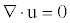(1)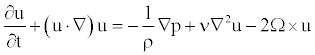(2)

where Ω is the angular velocity, and p is the modified pressure which includes the centrifugal effect.

Taking the curl of Equatation. (2) and substituting Equatation. (1) into the resultant equation, the vorticity equation is derived. The vorticity equation, expressed in the non-dimensional form by using the diameter D0 and circulation Γ0 of a vortex ring at the initial time, is written as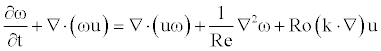(3)

where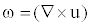is the vorticity, and k is the unit vector parallel to the rotating axis. The Reynolds number Re and the rotation number Ro are defined as.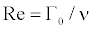(4)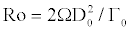(5)

Orthogonal decomposition of velocity

According to the Helmholtz theorem, the velocity u is the sum of the gradient of a scalar potential φ and the curl of a vector potential ψ :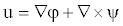(6)

The velocity calculated by (6) remains unaltered when any gradient of a scalar potential function is added to ψ . To remove this arbitrariness, the following solenoidal condition is imposed on ψ :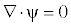(7)

Taking the curl of Equatation. (6) and substituting Equatation. (7) into the resultant equation, we obtain the following vector Poisson equation for ψ :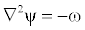(8)

Similarly, substituting Equatation. (6) into Equatation. (1) and recognizing that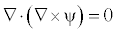, we obtain the Laplace equation for φ :

2ϕ = 0 (9)

Discretization of vorticity field into vortex elements

Once ψ and φ have been computed from Equatations. (8) and (9) respectively, the velocity u is calculated from Equatation. (6). The vorticity ω in Equatation. (8) is estimated from Equatation. (3). The VIC method discretizes the vorticity field into vortex elements, and calculates the distribution of ω by tracing the convection of each vortex element.

It is postulated that the position and vorticity for the vortex element p are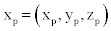and ωp, respectively. The Lagrangian form of the vorticity equation, Equatation. (3), is written as follows: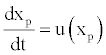(10)(11)

When the position and vorticity of a vortex element are known at time t , the values at t = t + Δt are computed from Eqs. (10) and (11). In the VIC method, the flow field is divided into computational grid cells to define ψ , φ and ω on the grids. If ω is defined at a position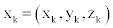the vorticity ω is assigned to xk , or a vortex element with ω is redistributed onto xk.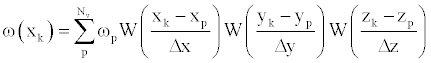(12)

where Nv is the number of vortex elements, and Δx , Δy and Δz are the grid widths. For the redistribution function W , the following equation is employed .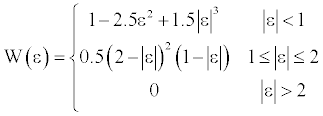(13)

Equation (13) was used for the simulations of time-developing free shear flows [11-13]. The DNS of a turbulent channel flow  and that of a jet issuing from a rectangular nozzle  were successfully performed with Equation. (13).

Discretization with staggered grid and correction of vorticity

When solving Equations. (8) and (9), staggered grids are used to ensure consistency between the discretized equations, and to prevent numerical oscillations of the solution . Figure 1 shows the staggered grid. The scalar potential φ is defined at the center of the grid. The velocity u is defined at the sides, while the vorticity ω and the vector potential ψ are defined on the edges.

In the VIC method, the vorticity field is discretized into vortex elements, and the field is expressed by superimposing the vorticity distributions around each vortex element. The superposition is performed by Equation. (12). The resulting vorticity field ωr does not necessarily satisfy the solenoidal condition. The authors' previous study  demonstrated that the curl of the velocity calculated from ωr yields a vorticity that satisfies the solenoidal condition. This correction method for the vorticity is employed in this simulation .

Numerical procedure

Given the flow at time t , the flow at t + Δt is simulated by the following procedure:

Calculate the time variation of ωp at every grid point from Equation. (11).

Calculate the convection of each vortex element from Equation. (10).

Calculate ω from Equatation. (12).

Calculate ψ from Equatation. (8).

Calculate φ from Equatation. (9).

Calculate u from Equatation. (6).

Correct the vorticity, or calculate the corrected vorticity from the curl of u.

#### Computational Conditions

At the initial time ( t* = 0 ), the vortex ring is expressed by giving the vorticity component ωφ normal to the cross-section of the vortex core. This study gives the vorticity distribution employed in the simulations of a vortex ring on a non-rotating field by Stanway et al.  and Wee-Ghoniem . The azimuthal vorticity ωφ is as follows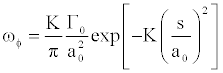(14)

where Γ0 is the circulation and K=2.241822/4. a0 and s are the core radius and the distance from the center of the vortex core respectively as shown in Figure 2a.

The origin of coordinates is set at the center of the vortex ring. The z-axis is parallel to the convection direction of the vortex ring. The angle between the radius vector r and the x-axis is denoted by ϕ . The contours of ωφ on the x-z section at y=0 are shown in Figure 2b.

Figure 3 shows the vortex ring at t* = 0 , where the iso-surface of the azimuthal vorticity ωφ,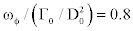, is presented. The computational domain of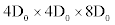is also shown in Figure 3. The boundary behind the vortex ring locates at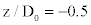. The number of grids is 80×80×160 . The computational domain rotates with a constant angular velocity Ω around the central axis (z-axis) of the vortex ring.

The computational conditions are listed in Table 1. The cases for the rotation number R0 of 0 ≤ R0 ≤ 0.2 are simulated at the core diameter of 2a0 /D0 = 0.35 and the Reynolds number of 500 Γ0 /ν = . As the vortex ring convects in quiescent fluid, φ is set at zero in the computational domain, and the solution of Equatation. (9) can be omitted. A slip condition is imposed on the boundaries. For example, the conditions at x / D0 = ± 2 are expressed as: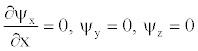(15)

 Core diameter;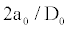0.35 Reynolds number;500 Rotation number;0, 0.08, 0.16, 0.2 Computational domain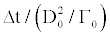Number of grids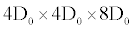Time increment;0.0125

Table 1: Computational conditions.

Four planes passing through the central axis of the vortex ring are set as shown in Figure 3. The strength of the vortex ring or the circulation Γ is calculated on the planes by the following equation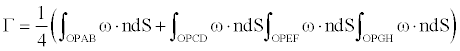(16)

where n is the unit vector normal to the plane.

Since the abovementioned four planes include the cross-section of the vortex core, the locations, at which the velocity falls to zero, exist on each plane. This simulation regards the locations as the center of the vortex core. The coordinates of the location on each plane are averaged to detect the diameter D and z-coordinate zv of the vortex ring.

#### Results and Discussion

Behavior of vortex ring on non-rotating field (R0=0)

The behavior of the vortex ring on the non-rotating filed (Ro=0) is shown in Figure 4. The azimuthal vorticity φ ω representing the vortex ring is presented with the iso-surface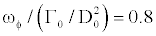at four time points. The vortex ring convects along the central axis (z-axis) with maintaining its axisymmetric vorticity distribution. The strength reduces with the passage of time owing to the viscous effect.

Figure 5 shows the time evolution of ωφ on the x-z cross-section at y=0. The vortex core is circular at t* = 0 as found from Figure 2b. But it is stretched along the central axis (z-axis) at t* = 6.25 , becoming ellipsoidal. In the rear end of the vortex core, the contours are axially elongated. The vorticity decreases with the passage of time at t* ≥ 6.25 . Such vorticity distribution coincides with the simulation by Wee and Ghoniem  at the same condition as this study. One can confirm the validity of the present simulation method.

The time-evolution characteristics of the vortex ring are shown in Figure 6. The circulation Γ / Γ0 decreases as the time passes. This is attributable to the viscous effect, and the decrement remains unaltered at t* ≥ 2.5 . The convection velocity also lowers with the passage of time because of the reduction of the circulation, as found from the time variation of the convection distance zv / D0. The diameter D / D0 increases as the time passes.

Behavior of vortex ring on rotating field (R0=0.2)

The behavior of the vortex ring at the rotation number R0=0.2 is shown in Figure 7, where the azimuthal vorticity ωφ that presents the vortex ring is plotted by the iso-surface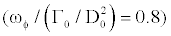in gray. The vortex ring convects straight along the rotating axis (z-axis) with maintaining its axisymmetric vorticity distribution. When compared with the result on the non-rotating filed (R0=0) shown in Figure 4, ωφ decreases markedly with the passage of time at t* ≥12.5 . The iso-surface disappears at t* = 25 . These are caused by a secondary vortex ring, the azimuthal vorticity of which is of opposite sign to that of the vortex ring, occurs at the head of the vortex ring, and accordingly it interacts with the vortex ring as mentioned later. In Figure 7, the vorticity component in the axial (z) direction, ωz is superimposed by the isosurface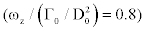in yellow. Though the axial vorticity ωz does not appear in the non-rotating condition (R0=0), it occurs locally around the central axis near the vortex ring at t* = 6.25 in the case of R0=0.2. Similar vorticity field was also numerically simulated by Verzicco et al.  at Re=1476 and R0=0.4. The azimuthal vorticity ωz is composed of the azimuthal velocity uϕ induced by the Coriolis force as discussed later. The streamwise longitudinal vortex, represented by ωz , is axisymmetric, and it is elongated in the axial (z) direction with the convection of the vortex ring. The strength of ωz decreases as the passage of time at t* ≥12.5 . The vorticity region of ωφ>0 corresponds to the vortex ring. The region of ωφ > 0 also occurs due to the rotation of the flow field. The iso-surface of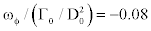is shown in blue. Such secondary vortex ring appears around the central axis at the head of the vortex ring when t* = 2.5 , and similar vortex ring also occurs in the rear of the vortex ring at t* = 3.75 . These secondary vortex rings are axisymmetric. In the study of Verzicco et al.  at Re=738 and R0=0.4, the secondary vortex ring at the head of the vortex ring was simulated. When t* ≥12.5 , the absolute value of the vorticity for the vortex ring decreases markedly with the passage of time. However, the streamwise longitudinal vortex and the secondary vortex rings, which are induced by the field rotation, less attenuate, and their iso-surfaces exist even at t* = 25 .

Figure 7: Time-evolution of azimuthal vorticity ωφ and axial vorticity ωz at R0=0.2 (Gray;, Blue;, Yellow;The time evolution of the distribution for the azimuthal velocity uϕ on the x-z section at y=0 is shown in Figure 8. The contours for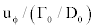are plotted, where the contours of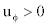and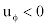are plotted in red and blue respectively. The region ofindicates that the flow direction coincides with the rotating direction of the field. The center of the vortex core is also indicated by the symbol +. The flow withappears around the central axis and behind the vortex ring. The velocity constitutes the axial vorticity ωz shown in Figure 7. The flows withare observed outside of the vortex cores and at the head of the vortex ring. The absolute value of the velocity is smaller.

Figure 8: Time-evolution of azimuthal velocity uø at R0=0.2 (Interval of contour lines Δuø is 0.01. Red and blue lines denote positive and negative values respectively).

The appearance of the azimuthal velocity uϕ shown in Figure 8 is caused by the Coriolis force. In the non-rotating condition (R0=0) at t* = 2.5 , the velocity distributes on the x-z section at y=0 as shown in Figure 9a. The planes at x < 0 and x > 0 are denoted by A and B, respectively. If the angle between the velocity u and the rotating axis (z-axis) is denoted by θ as shown in Figure 9b and c the flow withis induced by the Coriolis force on the planes A and B in the case of 0 <θ <π . Such condition for θ is satisfied by the flow toward the central (rotating) axis. It is confirmed that the condition is satisfied in the region ofshown in Figure 8. It should be noted that flow toward the central axis occurs behind the vortex cores, and the flow withexists there as found in Figure 8. This is caused by the fact that the flow withinduced at the head of the vortex ring is transported to the rear part due to the vortex motion of the vortex ring.

Figure 10 shows the velocity distribution on the x-y section perpendicular to the convection direction of the vortex ring. The results on the section 0.4D0 behind the vortex core are depicted. A circle passing through the vortex cores is superimposed. The diameter D corresponds to the diameter of the vortex ring. The flow withtoward the rotating direction of the flow field is observed around the central axis, and the small-scale flow withtoward the opposite direction is found outside of the vortex cores.

The secondary vortex rings having the negative azimuthal vorticity ωϕ are induced due to the rotation of the flow field as shown in Figure 7. The generation mechanism can be explained with the use of the vorticity equation for ωϕ: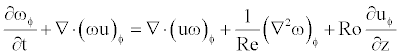(17)

One can find from Equatation. (17) that the azimuthal vorticity ωϕ is induced in the region where the azimuthal velocity uϕ varies in the convection direction of the vortex ring (z-direction). The velocity uϕ decreases markedly in the z-direction around the central axis at the head of the vortex ring as discovered in Figure 8. Figure 11 shows the distribution of ωϕ on the x-z section at y=0, where the contours of ωϕ < 0 are plotted in blue. Around the central axis at the head of the vortex ring or in the abovementioned region of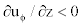the region of ωϕ < 0 or the secondary vortex ring is found. According to Figure 8, the region ofalso exists in the rear of the vortex ring. In this region, the secondary vortex ring with ωϕ < 0 also appears at 5 ≤ t* ≤12.5 shown in Figure 11. As the absolute value of the velocity gradient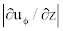is smaller than that at the head of the vortex ring, the strength of the secondary vortex ring is lower.

Figure 11: Time-evolution of azimuthal vorticity ωφ on x-z plane at Ro=0.2 (Interval of contour lines Δωφ is 0.08. Red and blue lines denote positive and negative values respectively).

Effect of R0 on behavior of vortex ring

The iso-surfaces of the vorticity components ωϕ and ωz at R0=0.08 and 0.16 are shown in Figure 12, where the results at t* =12.5 and 25 are plotted. When compared with the results at R0=0.2 shown in Figure 7, the reduction of the strength for the vortex ring becomes larger as R0 increases. The scale of the streamwise longitudinal vortex around the central axis and that of the secondary vortex rings become larger with the increase of R0.

Figure 12: Azimuthal vorticity ωφ and axial vorticity ωz at R0=0.08 and 0.16 (Gray;, Blue;, Yellow;Figure 13 shows the distribution of the axial velocity uz on the central axis, where the results at five time points for R0=0 and 0.2 are plotted. When R0=0.2, uz lessens greatly, demonstrating the reduction of the strength for the vortex ring due to the field rotation. The position, where uz takes its maximum value, corresponds to the center of the vortex core. When R0=0.2, uz decreases markedly at the head of the vortex core. This is because the secondary vortex ring with ωϕ < 0 and uz < 0 exists there.

The time-evolution characteristics of the vortex ring are shown in Figure 14. The decrement of the circulation Γ becomes larger with the increase of R0. The marked decrement occurs at 2.5 ≤ t* ≤12.5 . This is because the velocity around the vortex ring is higher, and accordingly the Coriolis force affects more the vortex ring. The convection velocity of the vortex ring also lowers due to the reduction of Γ . The diameter D increases more with the increment of R0. Because the secondary vortex ring with ωϕ < 0 appears at the head of the vortex ring as shown in Figure 11, and therefore it pushes the region with the positive azimuthal vorticity ωϕ > 0 in the radially outer direction.

Time evolution of helicity

The helicity H ( = u.ω ) is zero on the non-rotating field. But it takes the finite values on the rotating field. Calculating the helicity H at R0=0.2, it evolves with the passage of time as shown in Figure 15, where the iso-surfaces of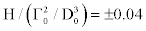are plotted. The positive helicity appears around the central axis behind the vortex ring. This presents the streamwise longitudinal vortex composed of the positive axial vorticity ωz induced by the Coriolis force and the positive axial velocity uz in the wake of the vortex ring. The negative helicity distributes along the vortex core. This presents the swirling flow composed of the negative azimuthal velocity vϕ induced by the Coriolis force and the positive azimuthal vorticity ωϕ of the vortex ring.

Figure 15: Time-evolution of helicity at R0=0.2 (Gray and blue isosurfaces denote and -0.04 respectively).

Figure 16 shows the streamlines at t* =12.5 in the case of R0=0.2. One can reconfirm the streamwise longitudinal vortex around the central axis behind the vortex core (the positive helicity) and the swirling flow along the vortex core (the negative helicity).

The iso-surfaces of the helicityat R0=0.08 and 0.16 are shown in Figure 17. The absolute value of the helicity decreases with the decrease of R0, and the streamwise longitudinal vortex and swirling flow induced by the field rotation reduce their scales.

Figure 17: Effect of Ro on helicity at t*=25 (Gray and blue iso-surfaces denoteand -0.04 respectively).

#### Conclusion

The behavior of a vortex ring on a field rotating with a constant angular velocity Ω is simulated. The vortex ring convects along the rotating axis of the field. The vortex in cell (VIC) method is employed for the simulation. The behavior at the rotation number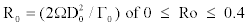is simulated when the Reynolds number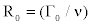is 500, where Γ0 and D0 are the circulation and diameter of the vortex ring at the initial time respectively. The results are summarized as follows:

(1) The vortex ring convects straight with maintaining its axisymmetric geometry in the same way on the non-rotating field. But the decrements of the vorticity and convection velocity with the passage of time become larger with the increment of R0. This is because a secondary vortex ring, the vorticity of which is of opposite sign to the vortex ring, appears at the head of the vortex ring, and accordingly it interacts with the vortex ring.

(2) The circumferential flow with the velocity uϕ > 0 toward the same direction as the rotating field occurs due to the Coriolis force around the central axis (rotating axis) behind the vortex ring. This demonstrates that the rotation yields the axial vorticity ωz. The wake of the vortex ring and ωz form the streamwise longitudinal vortex around the central axis.

(3) The circumferential flow with the azimuthal velocity uϕ < 0 toward the opposite direction of the rotating field appears owing to the Coriolis force outside of the vortex core. The absolute value of the velocity is smaller than that of the flow around the central axis. The vorticity of the vortex ring and uϕ form the swirling flow along the vortex core.

(4) The secondary vortex rings occur at the head and in the rear of the vortex ring. The vorticity has the opposite sign to that of the vortex ring. The secondary vortex rings are induced by the fact that the Coriolis force acts on the circumferential flow with the velocity uϕ caused by the field rotation.

(5) The streamwise longitudinal vortex around the central axis and the swirling flow along the vortex core become larger with the increment of Ro. The increment in the diameter of the vortex ring with the passage of time is also larger. Because the secondary vortex rings appearing at the head of the vortex ring extrudes the vortex ring to the outside.

Nomenclatures

a: core radius of vortex ring

D: diameter of vortex ring

H: helicity = u.ω

k: unit vector parallel to rotating axis

Nv: number of vortex elements

n: unit normal vector

P: pressure

Re: Reynolds number =Γ0 / ν

R0: rotation number =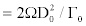S: distance from center of vortex core

t: time

t*: non-dimensional time =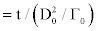u: velocity

W: redistribution function of vorticity

x, y, z : orthogonal coordinates

zv : z-coordinate of center for vortex core

Γ : circulation

Δt : time increment

Δx,Δy,Δz : width of computational grid cell

ν : kinematic viscosity

ρ : density

φ : scalar potential

ϕ : angle between radius vector and x-axis

ψ : vector potential

Ω : angular velocity

ω : vorticity = ∇× u

Subscripts

0 : initial value

x, y, z : components in directions of x, y and z

ϕ : azimuthal component (Table 1).

#### References

Select your language of interest to view the total content in your interested language

### Article Usage

• Total views: 13151
• [From(publication date):
March-2015 - Jan 28, 2020]
• Breakdown by view type
• HTML page views : 9329Can't read the image? click here to refresh